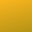#Abstracting Words and Numbers

We informally compare the layering of concepts in both mathematics and computaton. We observe that with each layer an abstraction is formed that normalizes a useful manipulation.

# Mathematics

Numbers come from counting or measuring. We can add or multiply numbers or even invert these operations to get subtraction or division. We can name a number and then further manipulate it: x, x·x, x².

We recognize some systems of operations are useful such as the polynomial: a·x² + b·x + c. The variables a, b and c are the expressive part here and held firm while considering all possible values of x.

We consider systems of equations expressed in orderly collections of polynomial coefficients, which we metaphorically call a matrix.

$\begin{matrix} a₀ & a₁ & a₂ \\ b₀ & b₁ & b₂ \\ c₀ & c₁ & c₂ \end{matrix}$

# Computation

Words come from phonetic sequences of letters which may or may not have meaning in the world outside of computation. A list of words, {alpha beta gamma}, can express an operation if alpha is recognized as naming a replacement.

Lambda, sometimes written λ, expresses the replacement of arguments into a list.

{lambda {beta} alpha beta gamma}

Lamba plus a small number of additional operations can be nested to perform arbitrary computations. For example.

{def foo {lambda {beta} alpha beta gamma}}

Where def means define the next word, foo, as expressing the operation defined by the following words.

# Hypertext

Words have their usual meanings drawn from natural language. Paragraphs are words assembled into statements that expresses a thought.

A page has a title made from words and a story made from paragraphs.

A title enclosed in square brackets will display as a link when clicked exposes the story by that name. From this arbitrarily complex paths through the stories are made possible.

# Comparison

Each system offers a mechanism for naming structures found in the domain of discourse. The systems differ in when the abstractions so created are applied.

In mathematics the abstractions hold for all time.

In computation the abstractions apply in sequence.

In hypertext the abstractions apply when clicked.

Systems are easily built that employ some combination of these approaches exploiting each to their advantage. Notations become slightly more complex but also much more expressive and convenient.